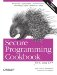# Finding the Greatest or Least Value in a Container

Problem

You want to find the maximum or minimum value in a container.

Solution

Example 11-2 shows how to find the minimum and maximum elements in a container by using the functions max_element and min_element found in the header. These functions return iterators that point to the first occurence of an element with the largest or smallest value, respectively.

Example 11-2. Finding the minimum or maximum element from a container

```#include
#include
#include

using namespace std;

int getMaxInt(vector& v) {
return *max_element(v.begin( ), v.end( ));
}

int getMinInt(vector& v) {
return *min_element(v.begin( ), v.end( ));
}

int main( ) {
vector v;
for (int i=10; i < 20; ++i) v.push_back(i);
cout << "min integer = " << getMinInt(v) << endl;
cout << "max integer = " << getMaxInt(v) << endl;
}```

The program in Example 11-2 produces the following output:

```min integer = 10
max integer = 19```

Discussion

You may have noticed the dereferencing of the return value from the calls to min_element and max_element. This is because these functions return iterators and not actual values, so the results have to be dereferenced. You may find it a minor inconvenience to have to dereference the return type, but it avoids unnecssarily copying the return value. This can be especially significant when the return value has expensive copy semantics (e.g., large strings).

The generic algorithms provided by the standard library are obviously quite useful, but it is more important for you to be able to write your own generic functions for getting the minimum and maximum value from a container. For instance, let's say that you want a single function which returns the minimum and maximum values by modifying reference parameters instead of returning them in a pair or some other structure. This is demonstrated in Example 11-3.

Example 11-3. Generic function for returning the minimum and maximum value

```#include
#include
#include

using namespace std;

template
void computeMinAndMax(Iter_T first, Iter_T last, Value_T& min, Value_T& max) {
min = *min_element(first, last);
max = *max_element(first, last);
}

int main( ) {
vector v;
for (int i=10; i < 20; ++i) v.push_back(i);
int min = -1;
int max = -1;
computeMinAndMax(v.begin( ), v.end( ), min, max);
cout << "min integer = " << min << endl;
cout << "max integer = " << max << endl;
}```

In Example 11-3, I have written a computeMinAndMax function template which takes two template parameters, one is the type of the iterator and the other is the type of the minimum and maximum values. Because both template parameters are also function parameters, the C++ compiler is able to deduce the two separate types, Iter_T and Value_T, just as I demonstrated in Recipe 11.1. This saves me from having to specify the template parameters explicitly like:

`compute_min_max::iterator, int>(...)`

The min_element and max_element functions work by using operator< to compare the values referenced by the iterators. This means that if an iterator does not reference a type that supports comparison through the less-than operator, a compiler error will result. The min_element and max_element functions, however, can be used with a user-defined comparison functor, i.e., a function pointer or a function object.

## Functors

Many STL algorithms accept user-defined function objects or pointers. Collectively, these are known as functors. Sometimes in the literature, the term "function object" is used interchangeably with the term functor, but I've reserved the use of function object for refering specifically to instances of classes or structs that overload operator( ).

Of the two options, which one should you use? In the majority of cases, a function object is more efficient because most compilers can more easily inline a function object.

Another reason for using a function object is that it can have a state. You can pass values to its constructor, which it stores in its fields for use later on. This gives function objects an expressive equivalency as with the concept of closures found in other programming languages.

Finally, function objects can be defined within another function or class. Function pointers have to be declared in a namespace scope.

The specific kind of functor needed by min_element and max_element is one that takes two values of the type referenced by the iterator and returns a boolean value if one is less than the other. A functor which returns a boolean value is known as a predicate. Consider for instance the case of finding the greatest element of a set of user defined type in Example 11-4.

Example 11-4. Finding the maximum element for user-defined types

```#include
#include
#include

using namespace std;

struct ChessPlayer {
ChessPlayer(const char* name, int rating)
: name_(name), rating_(rating)
{ }
const char* name_;
int rating_;
};

struct IsWeakerPlayer {
bool operator( )(const ChessPlayer& x, const ChessPlayer& y) {
return x.rating_ < y.rating_;
}
};

int main( )
{
ChessPlayer kasparov("Garry Kasparov", 2805);
ChessPlayer anand("Viswanathan Anand ", 2788);
ChessPlayer topalov("Veselin Topalov", 2788);
vector v;
v.push_back(kasparov);
v.push_back(anand);
v.push_back(topalov);
cout << "the best player is ";
cout << max_element(v.begin( ), v.end( ), IsWeakerPlayer( ))->name_;
cout << endl;
}```

The program in Example 11-4 produces the following output:

`the best player is Garry Kasparov`

In Example 11-4 I have shown how to provide max_element with a custom predicate. The predicate is the function object IsWeakerPlayer.

Another alternative to pasing a user-defined predicate in Example 11-4 is to override operator< for the ChessPlayer struct. This works fine for the specific case of the example, but it presumes that the most important way to sort players is by rating. It may be that sorting by name is more prevalent. Since choosing one method of sorting over another in this case is an arbitrary choice, I prefer to leave operator< undefined.Secure Programming Cookbook for C and C++: Recipes for Cryptography, Authentication, Input Validation & More
ISBN: 0596003943
EAN: 2147483647
Year: 2006
Pages: 241